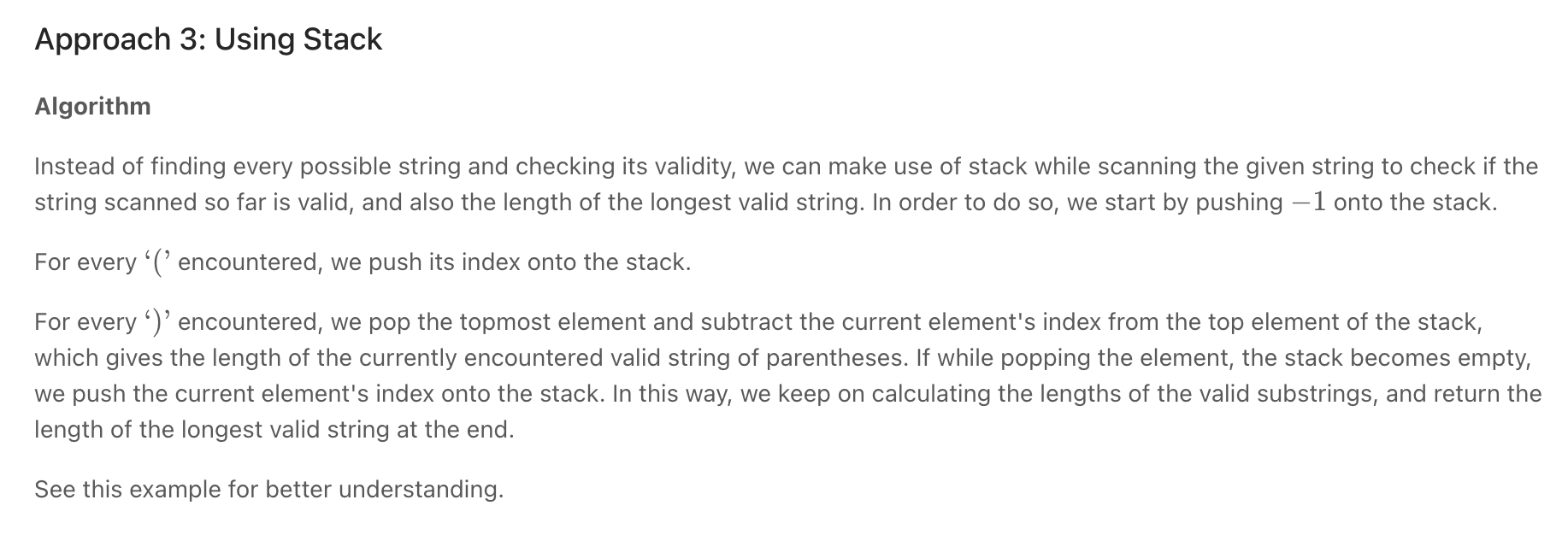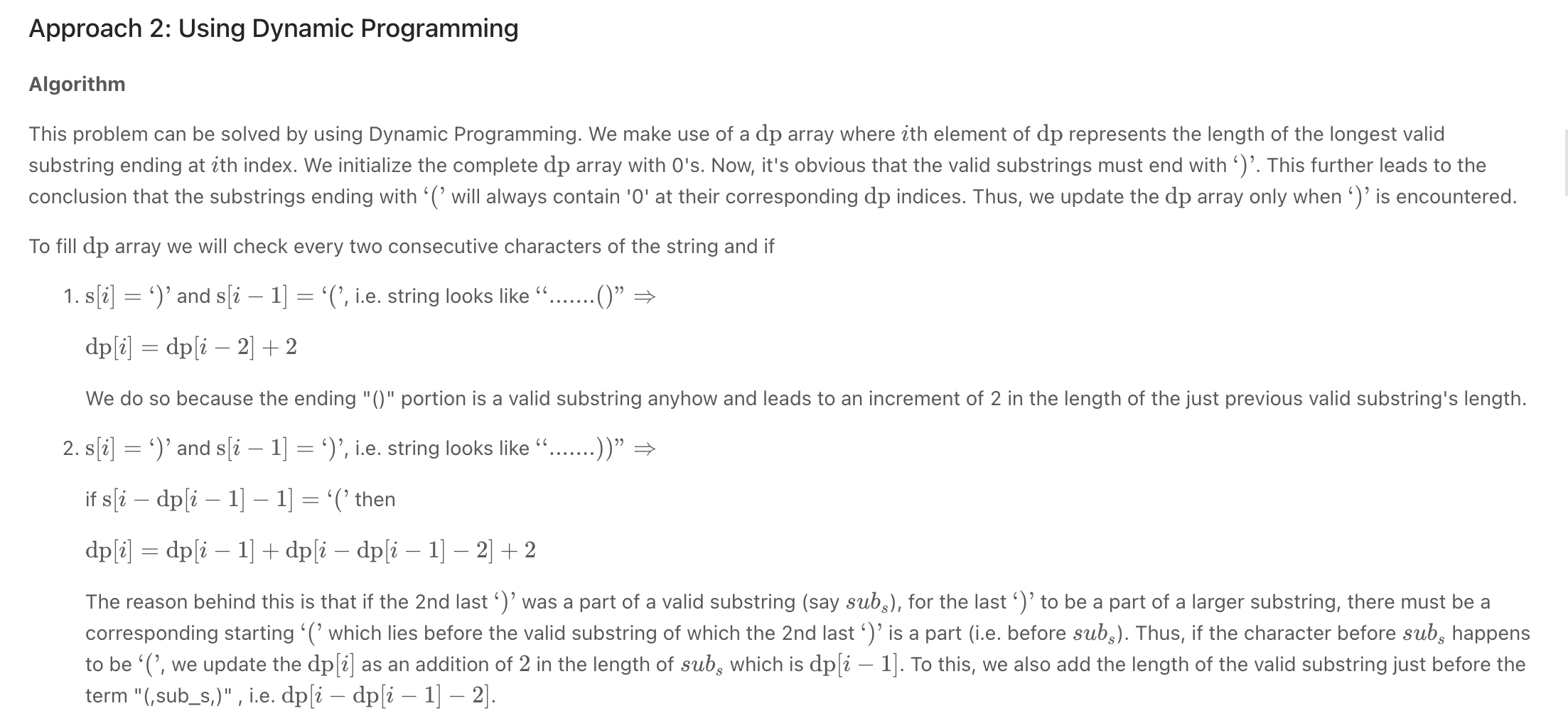# Parenthesis

• Valid Parentheses / Balanced Brackets

"""
Valid Parentheses / Balanced Brackets

Given a string s containing just the characters '(', ')', '{', '}', '[' and ']', determine if the input string is valid.
An input string is valid if:
Open brackets must be closed by the same type of brackets.
Open brackets must be closed in the correct order.

https://leetcode.com/problems/valid-parentheses/
https://www.algoexpert.io/questions/Balanced%20Brackets
"""

class Solution(object):
def isValid(self, s):

myStack = []

match = {
"(": ")",
"[": "]",
"{": "}"
}

for par in s:
if par == "(" or par == "{" or par == "[":
myStack.append(par)

elif len(myStack) == 0 or match[myStack.pop()] != par:
return False

return len(myStack) == 0

def balancedBrackets(string):
opening_brackets = "([{"
matching_brackets = {")": "(", "]": "[", "}": "{"}
stack = []
for char in string:

if char not in matching_brackets and char in opening_brackets:  # opening bracket
stack.append(char)

# closing brackets
elif char in matching_brackets and(not stack or matching_brackets[char] != stack.pop(-1)):
return False

return len(stack) == 0

print(balancedBrackets("([])(){}(())()()"))
print(balancedBrackets("([])(){}(()))()()"))

• Minimum Add to Make Parentheses Valid

"""
921. Minimum Add to Make Parentheses Valid

A parentheses string is valid if and only if:
It is the empty string,
It can be written as AB (A concatenated with B), where A and B are valid strings, or
It can be written as (A), where A is a valid string.
You are given a parentheses string s.
In one move, you can insert a parenthesis at any position of the string.
For example, if s = "()))", you can insert an opening parenthesis to be "(()))" or a closing parenthesis to be "())))".
Return the minimum number of moves required to make s valid.

Example 1:

Input: s = "())"
Output: 1
Example 2:

Input: s = "((("
Output: 3
Example 3:

Input: s = "()"
Output: 0
Example 4:

Input: s = "()))(("
Output: 4

"""

class Solution:
invalid_closing = 0
invalid_opening = 0

# invalid closing
opening_remaining = 0
for char in s:
if char == "(":
opening_remaining += 1
else:
if opening_remaining > 0:
opening_remaining -= 1
else:
invalid_closing += 1

# invalid closing
closing_remaining = 0
for idx in reversed(range(len(s))):
if s[idx] == ")":
closing_remaining += 1
else:
if closing_remaining > 0:
closing_remaining -= 1
else:
invalid_opening += 1

return invalid_opening + invalid_closing

• Longest Valid Parentheses **Screen Recording 2021-10-17 at 11.27.12.movScreen Recording 2021-11-03 at 18.23.28.mov

"""
Longest Valid Parentheses:

Given a string containing just the characters '(' and ')',
find the length of the longest valid (well-formed) parentheses substring.

https://leetcode.com/problems/longest-valid-parentheses/
https://paulonteri.notion.site/Parenthesis-b2a79fe0baaf47459a53183b2f99115c
"""

class Solution:
def longestValidParentheses(self, s: str):
if not s:
return 0

opening_brackets = 0
longest_so_far = *(len(s)+1)
for idx, char in enumerate(s):
# opening brackets
if char == '(':
opening_brackets += 1

# closing brackets
else:
if opening_brackets <= 0:
continue
opening_brackets -= 1
length = 2  # create streak

# # "()"
if s[idx-1] == "(" and idx > 1:
# add what is outside the brackets. Eg: (())() - at the last idx
length += longest_so_far[idx-2]

# #"))"
elif s[idx-1] == ")":
# continue streak
length += longest_so_far[idx-1]
# add what is outside the brackets. Eg: ()(()) - at the last idx
if idx-length >= 0:
length += longest_so_far[idx-length]

longest_so_far[idx] = length

return max(longest_so_far)

"""
"((())())"
['(', '(', '(', ')', ')', '(', ')', ')']
[  0,  1,    2,  3,   4,   5,   6,   7]

idx,res,c_l,stack
0    0   0  
1    0   0  [0,0]
2    0   0  [0,0,0]
3    2   2  [0,0]
4    4   4  
5    4   0  [4,0]
6    6   6  
7    8   8  []

"""

class Solution__:
def longestValidParentheses(self, s: str):
"""
Whenever we see a new open parenthesis, we push the current longest streak to the prev_streak_stack.
and reset the current length
Whenever we see a close parenthesis,
If there is no matching open parenthesis for a close parenthesis,
reset the current count.
else:
we pop the top value, and add the value (which was the previous longest streak up to that point)
to the current one (because they are now contiguous)
and add 2 to count for the matching open and close parenthesis.

Use this example to understand "(()())"
"""
res = 0

prev_streak_stack, curr_length = [], 0
for char in s:

# # saves streak that might be continued or broken by the next closing brackets
if char == '(':
prev_streak_stack.append(curr_length)  # save prev streak
curr_length = 0  # reset streak

# # create/increase or reset streak
elif char == ')':

if prev_streak_stack:
curr_length = curr_length + prev_streak_stack.pop() + 2
res = max(res, curr_length)

else:
curr_length = 0

return res

"""

"""

class Solution_:
def longestValidParentheses(self, s: str):
"""
use stack to store left most invalid positions (followed by opening brackets)
note that an unclosed opening brackets is also invalid
"""
result = 0

# stack contains left most invalid positions
stack = [-1]

for idx, bracket in enumerate(s):
if bracket == "(":
stack.append(idx)

else:
stack.pop()

# the top of the stack should now contain the left most invalid index
if stack:
result = max(result, idx-stack[-1])
# if not, the element removed was not an opening bracket but the left most invalid index
# which means that this closing bracket is invalid and should be added to the top of the stack
else:
stack.append(idx)

return result


Maximum Nesting Depth of Two Valid Parentheses Strings - LeetCode